Tamilnadu State Board New Syllabus Samacheer Kalvi 11th Computer Science Guide Pdf Chapter 8 Iteration and Recursion Text Book Back Questions and Answers, Notes.

## Tamilnadu Samacheer Kalvi 11th Computer Science Solutions Chapter 8 Iteration and Recursion

### 11th Computer Science Guide Iteration and Recursion Text Book Questions and AnswersPart I

Question 1.
A loop invariant need not be true.
a) at the start of the loop
b) at the start of each iteration
c) at the end of each iteration
d) at the start of the algorithm
d) at the start of the algorithm

Question 2.
We wish to cover a chessboard with dominoes, I I 1 the number of black squares and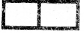the number of white squares covered by dominoes, respectively, placing a domino can be modeled by
a) b ; = b + 2
b) w := w + 2
c) b, w : = b + 1, w + 1
d) b : = w
d) b : = wQuestion 3.
If m x a + n x b is an invariant for the assignment a, b : -a + 8, b + 7, the values of m and n are
a) m = 8, n = 7
b) m – 1, n = -8
c) m = 7, n = 8
d) m – 8, n = -7
b) m – 1, n = -8

Question 4.
Which of the following is not an invariant of the assignment? m, n ; = m + 2, n + 3.
a) m mod 2
b) n mod 3
c) 3 x m – 2 x n
d) 2 x m – 3 x n
d) 2 x m – 3 x n

Question 5.
If Fibonacci number is defined recursively as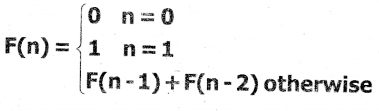to evaluate F(4), how many times F( ) is applied?
a) 3
b) 4
c) 8
d) 9
a) 3Question 6.
Using this recursive definition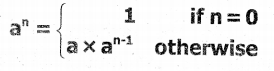how many multiplications are needed to calculate a10?
a) 11
b) 10
c) 9
d) 8
c) 9

Part – II

Question 1.
What is an invariant?
An expression involving variables, which remain unchanged by an assignment to one of these variables is called an invariant of the assignment.

Question 2.
Define a loop invariant.
Each time the loop body is executed, the variables which remains unchanged by the execution of the loop body is called the loop invariant.

Question 3.
Does testing the loop condition affect the loop invariant? Why?
No, the loop condition does not affect the loop invariant. Because the loop invariant is true at four points.

1. At the start of the loop.
2. At the start of each iteration.
3. At the end of each iteration.
4. At the end of the loop.Question 4.
What is the relationship between loop invariant, loop condition, and the input-output recursively?

• Establish the loop invariant at the start of the loop.
• The loop body should so update the variables as to progress toward the end and maintain the loop invariant, at the same time.
• When the loop ends, the termination condition and the loop invariant should establish the input-output relation.

Question 5.
What is recursive problem-solving?
Recursion is a method of solving problems that involves breaking a problem down into smaller and smaller subproblems until the user gets into a small problem that can be solved trivially. Usually, recursion involves a function calling itself. While it may not seem like much on the surface, recursion allows us to write elegant solutions to problems that may otherwise be very difficult to program.

Question 6.
Define factorial of a natural number recursively.
factoriai(n)
— inputs: n
— outputs: f
if n = 0
f = 1
else
f = n x factorial(n -1) → Recursive process
Example:
To calculate 5 factorial factorial(5)
= 5 x factorial(4)
= 5 x 4 x factorial(3)
= 5 x 4 x 3 x factorial(2)
= 5 x 4 x 3 x 2 x factorial (1) =5 x 4 x 3 x 2 x 1 = 120Part – III

Explain In Brief

Question 1.
There are 7 tumblers on a table, all standing upside down. You are allowed to turn any 2 tumblers simultaneously in one move. Is it possible to reach a situation when all the tumblers are right side up? (Hint: The parity of the number of upside-down tumblers is invariant).
Solution:
Let u – No. of tumblers right side up
v – No. of tumblers upside down
Initial stage : u = 0, v = 7 (All tumblers upside down)
Final stage output: u = 7, v = 0 (All tumblers right side up)

Possible Iterations:
(i) Turning both up side down tumblers to right side up
u = u + 2, v = v – 2 [u is even]

(ii) Turning both right side up tumblers to upside down.
u = u – 2, v = v + 2 [u is even]

(iii) Turning one right side up tumblers to upside down and other tumblers from upside down to right side up.
u = u + 1 – 1 = u, v = v + 1 – 1 = v [u is even]

Initially u = 0 and continues to be even in all three cases. Therefore u is always even. Invariant: u is even (i. e. No. of right side up tumblers are always even)
But in the final stage (Goal), u = 7 and v = 0 i. e. u is odd.
Therefore it is not possible to reach a situation where all the tumblers are right side up.Question 2.
A knockout tournament is a series of games. Two players compete in each game; the loser is knocked out (i.e. does not play anymore), the winner carries on. The winner of the tournament is the player that is left after all other players have been knocked out. Suppose there are 1234 players in a tournament. How many games are played before the tournament winner is decided?
Solution :
Suppose there are 2 players A and B competing in a knockout match, so the number of possible matches is – A Vs B
Suppose there are 3 players A, B and C competing in a knockout match, so the Number of possible matches is – 2.
A Vs B , and A/B( Whoever wins with plays with C) vs C.
Suppose there are 4 players A, B, C & D competing in a knockout match, so the Number of possible matches is – 3.
A Vs B , C Vs D A/B Vs C/D
Thus the General Observations is –
When there are 2 players – 1 Match
When there are 3 players – 2 Matches
When there are 4 players – 3 Matches
When there are n players – ( n – 1) Matches
Hence when there are 1234 players there will be 1233 matches.
The number of games in a single-elimination tournament is always 1 less than the number of players/teams.

Question 3.
King Vikramaditya has two magic swords. With one, he can cut off 19 heads of a dragon, but after that, the dragon grows 13 heads. With the other sword, he can cut off 7 heads, but 22 new heads grow. If all heads are cut off, the dragon dies. If the dragon has originally 1000 heads, can it ever die? (Hint: The number of heads mod 3 is invariant.)
No. of heads of dragon = 1000
Let n be the number of heads of the dragon at the initial state.

Case 1: King uses Sword 1
n : = n – 19 + 13 = n – 6 No. of heads are reduced by 6.

Case 2: King uses Sword 2
n : = n – 7 + 22 = n + 15
No. of heads are increased by 15.

Note:
In the above two cases, either 6 heads are removed or 15 heads added. Both 6 and 15 are multiples of 3.
Therefore repeating case 1 and case 2 recursively will either reduce or increase dragon heads in multiples of 3.
That is invariant is n mod 3.
If n mod 3 = 0 then there is a possibility that the dragon dies.
But 1000 is not a multiple of 3 1000 mod 3 = 1 ≠ 0
It is not possible to kill the dragon. The dragon never dies.Part IV

Explain In Detail

Question 1.
Assume an 8 x 8 chessboard with the usual coloring. “Recoloring” operation changes the color of all squares of a row or a column. You can recolor repeatedly. The goal is to attain just one black square. Show that you cannot achieve the goal. (Hint: If a row or column has b black squares, it changes by (|8 – b) – b |).
Solution:
Let us start with a normal coloured chessboard, with a number of black squares B=32 and the number of white squares W=32.
So W – B = 0, which is divisible by 4 and W + B = 64.
W-B = 0 mod 4

Whenever we change the colours of a row or column, we change the colour of 8 squares. Let this row (or column) have w white squares + b black squares w + b = 8 squares.

If this operation B increases (or decreases) by 2n, then W decreases (or increases) by 2n so that W + B = 64, but B – W will change by 4n and it will remain divisible by 4.
W – B = 0 mod 4

After every operation, “B – W mod 4” can have no other values.
But the required state has 63 white squares and 1 black square, so it requires
W-B = 63-1 = 62 = 2 mod 4 which is impossible.

Question 2.
Power can also be defined recursively as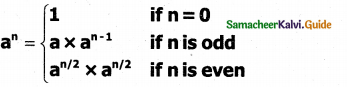Construct a recursive algorithm using this definition. How many multiplications are needed to calculate a10?
Recursive Algorithm
power(a,n)
— inputs: n is an integer, n ≥ 0
— outputs: an
if n = 0 → Base case
1
else
if(n%2 = 0)
a x power(a, an-1) → Recursion step for odd number else
a x power(an/2 x an/2) → → Recursion step for even number

To calculate a10
power(a, 10)
= a x power(a5 x a5)
= a x power(a, a4) x power(a, a4)
= a x a x power(a2 x a2) x power(a, a4)
= a x a x a x power(a, a0) x power(a, a0 ) x
power(a, a4)
= a x a x a x a x a x power(a2 x a2)
= a x a x a x a x a x a x a x power(a, a0) x a x power(a, a0)
=a x a x a x a x a x a x a x a x a x a
There are nine multiplications are needed.

Question 3.
A single-square-covered board is a board of 2n x 2n squares in which one square is covered with a single square tile. Show that it is possible to cover this board with triominoes without overlap.
Solution :
A triamine is an L-shaped tile formed with three adjacent squares.
Corner-covered board and triamine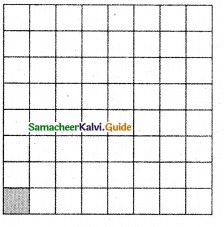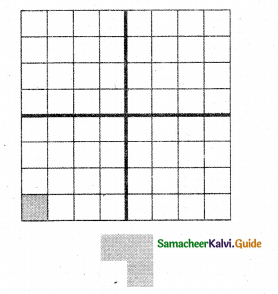Cover the corner-covered board with the L-shaped triominoes without overlap, Triominoes can be rotated as needed.
The size of the problem is n (board of size 2n x 2n). We can solve the problem by recursion. The base case is n = 1. It is a 2 x 2 corner-covered board. We can cover it with one triamine and solve the problem.

In the recursion step, divide the corner-covered board of size 2n x 2n into 4 sub-boards, each of size 2n-1 x 2n-1, by drawing horizontal and vertical lines through the center of the board. Place a triamine at the center of the entire board so as to not cover the corner-covered sub-board, as shown in the left-most board of the given figure below. Now, we have four corner-covered boards, each of size 2n-1 x 2n-1.
Recursive process of covering a corner-covered board of size 2 x 23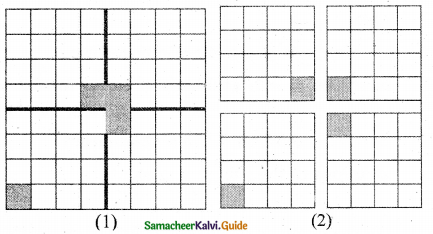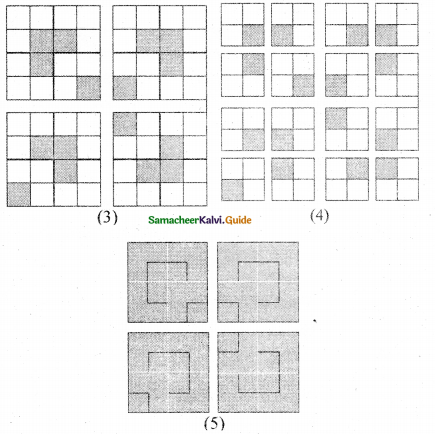We have 4 sub-problems whose size is strictly smaller than the size of the given problem. We can solve each of the sub-problems recursively. tile corner_covered board of size n
if n = 1 — base case
cover the 3 squares with one triominoe
else — recursion step
divide board into 4 sub_boards of size n – 1
place a triominoe at centre of the board
leaving out the corner_covered sub-board
tile each sub-board of size n-1

The resulting recursive process for covering a 23 x 23 corner-covered board is illustrated in the above Figure.### 11th Computer Science Guide Iteration and Recursion Additional Questions and Answers

Part I

Question 1.
………………… is an algorithm design technique, closely related to induction.
(a) Iteration
(b) Invariant
(c) Loop invariant
(d) Recursion
(d) Recursion

Question 2.
Each time the loop body is executed, the variables are _________
a) updated
b) unchanged
c) neither A nor B
d) destroyed
a) updated

Question 3.
In a loop, if L is an invariant of the loop body B, then L is known as a …………………
(a) recursion
(b) variant
(c) loop invariant
(d) algorithm
(c) loop invariantQuestion 4.
_________ is more powerful than the iteration.
a) Recursion
b) Specification
c) Composition
d) None of these
a) Recursion

Question 5.
The unchanged variables of the loop body are…………………
(a) loop invariant
(b) loop variant
(c) condition
(d) loop variable
(a) loop invariant

Question 6.
_________ is a recursive solver case.
a) Base case
b) Recursion steps
c) Both A and B
d) None of these
c) Both A and B

Question 7.
If L is a loop variant, then it should be true at ………………… important points in the algorithm.
(a) 2
(b) 3
(c) 4
(d) 5
(c) 4Question 8.
In_________, the size of moot to a sub-problem must be strictly smaller than the size of the given input.
a) Recursion
b) Specification
c) Composition
d) None of these
a) Recursion

Question 9.
In an expression, if the variables have the same value before and after an assignment, then it is of an assignment.
(a) variant
(b) Invariant
(c) iteration
(d) variable
(b) Invariant

Question 10.
An expression involving variables, which remains unchanged by an assignment to one of these variables, is called _________ of the assignment.
a) an invariant
b) variant
c) neither A nor B
d) None of these
a) an invariantQuestion 11.
When the solver calls a sub solver, then it is called …………………
(a) Iterative call
(b) solver call
(c) recursive call
(d) conditional call
(c) recursive call

Question 12.
_________ Is an algorithm design technique
to execute the same action repeatedly.
a) iteration
b) recursion
c) Both iteration and recursion
d) None of these
c) Both iteration and recursion

Question 13.
Which of the following is updated when each time the loop body is executed?
(a) data type
(b) subprogram
(c) function
(d) variable
(d) variableQuestion 14.
Recursion is an algorithm design technique, closely related to _________
a) induction
b) introduction
c) intuition
d) None of these
a) induction

Part II

Question 1.
When the loop variant will be true?
The loop invariant is true before the loop body and after the loop body, each time.

Question 2.
How problems are solved using recursion?
Using recursion, we solve a problem with a given input, by solving the same problem with a part of the input and constructing a solution to the original problem from the solution to the partial input.

Question 3.
If L is a loop variant, then where it is true in the algorithm?
It is true in the following four points of an algorithm.

1. at the start of the loop.
2. at the start of each iteration.
3. at the end of each iteration.
4. at the end of the loop.

Part – III

Explain In Brief

Question 1.
Write a note on Recursion.
Recursion:
Recursion is another algorithm design technique, closely related to iteration, but more powerful. Using recursion, we solve a problem with a given input, by solving the same problem with a part of the input and constructing a solution to the original problem from the solution to the partial input.

Question 2.
Write the recursive algorithm for the length of a sequence.
The recursive algorithm for the length of a sequence
can be written as
length (s)
— inputs: s
— outputs : length of s
if s has one customer — base case
1
else
1 + length(tail(s)) — recursion step.Part IV

Explain In Detail

Question 1.
Explain loop Invariant in detail.
In a loop, if L is an invariant of the loop body B, then L is known as a loop invariant,
while C
— L
B
— L
The loop invariant is true before the loop body and after the loop body, each time. Since L is true at the start of the first iteration, L is true

at the start of the loop also (just before the loop). Since L Is true at the end of the last iteration, L is true when the loop ends also (just after the loop). Thus, if L is a loop variant, then it is true at four important points in the algorithm, as annotated in the algorithm and shown in Figure 3.1.

i) at the start of the loop (just before the loop)
ii) at the start of each iteration (before loop body)
iii) at the end of each iteration (after loop body)
iv) at the end of the loop (just after the loop)

i) — L, start of loop
while
C

ii) — L, start of iteration
B

iii) –L, end of Iteration

iv) — L, end of loop

The points where the loop invariant is true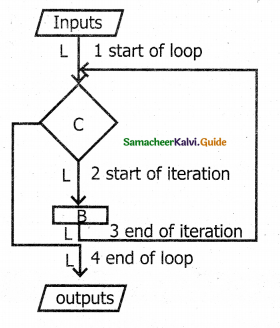Question 2.
Design an iterative algorithm to compute an.
Let us name the algorithm power(a, n).
For example,
power(10, 4) = 10000
power (5,3) = 125 .
power (2,5) = 32
Algorithm power(a, n) computes an by multiplying a cumulatively n times.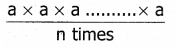The specification and the loop invariant are shown as comments.

power (a, n)
— inputs: n is a positive integer
— outputs: p = an
p, i := 1,0
while i ≠ n
— loop invariant: p = ai
p, i: = p x a, i + 1
The step by step execution of power (2, 5) is shown in the following Table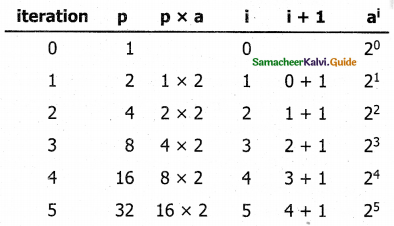Question 3.
Explain the outline of the recursive problem-solving technique.